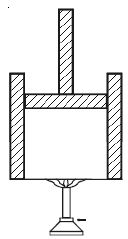Physics

# Explain First law of Thermodynamics

Let consider a gas inside a cylinder fitted with a movable friction-less piston. The walls of the cylinder are made up of non-conducting material and the bottom is made up of conducting material (Figure).Let the bottom of the cylinder be brought in contact with a hot body like burner. The entire heat energy given to the gas is not converted into work. A part of the heat energy is used up in increasing the temperature of the gas (i e) in increasing its internal energy and the remaining energy is used up in pushing the piston upwards (i.e.) in doing work. If ∆Q is the heat energy supplied to the gas, U1 and U2 are initial and final internal energies and ∆W is the work done by the system, then

∆Q = ∆W + (U2 – U1)

∆Q = ∆W + ∆U

Where, ∆U is the change in the internal energy of the system. Hence, the first law of thermodynamics states that the amount of heat energy supplied to a system is equal to the sum of the change in internal energy of the system and the work done by the system. This law is in accordance with the law of conservation of energy.